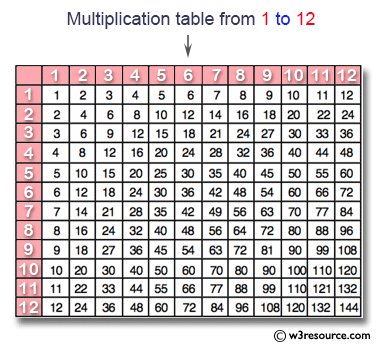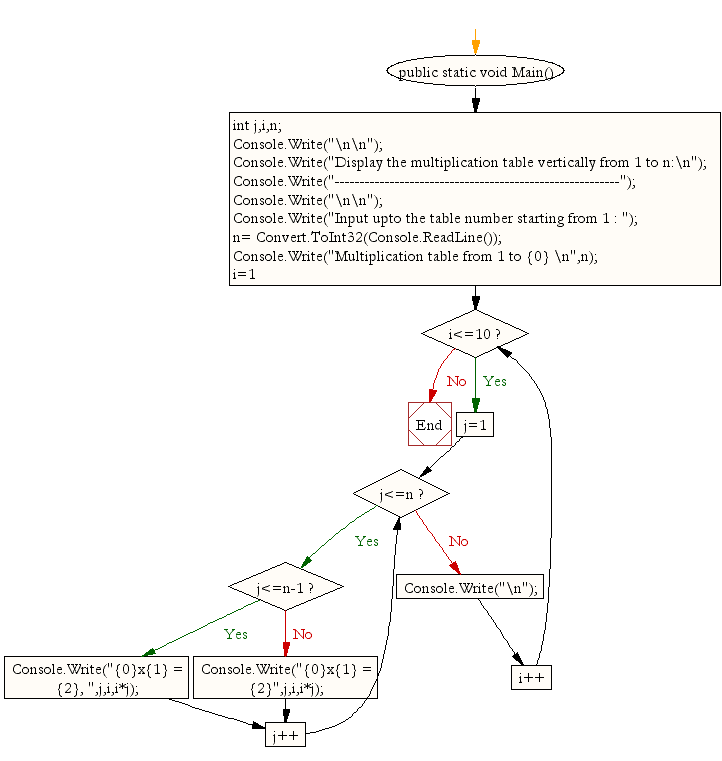﻿ C# - Display n number of multipliaction table vertically# C# Sharp Exercises: Display n number of multiplication table vertically

## C# Sharp For Loop: Exercise-7 with Solution

Write a program in C# Sharp to display the multiplication table vertically from 1 to n.

Pictorial Presentation:Sample Solution:

C# Sharp Code:

``````using System;
public class Exercise7
{
public static void Main()
{
int j,i,n;
Console.Write("\n\n");
Console.Write("Display the multiplication table vertically from 1 to n:\n");
Console.Write("---------------------------------------------------------");
Console.Write("\n\n");

Console.Write("Input upto the table number starting from 1 : ");
Console.Write("Multiplication table from 1 to {0} \n",n);
for(i=1;i<=10;i++)
{
for(j=1;j<=n;j++)
{
if (j<=n-1)
Console.Write("{0}x{1} = {2}, ",j,i,i*j);
else
Console.Write("{0}x{1} = {2}",j,i,i*j);

}
Console.Write("\n");
}
}
}```
```

Sample Output:

```Display the multiplication table vertically from 1 to n:
---------------------------------------------------------

Input upto the table number starting from 1 : 5
Multiplication table from 1 to 5
1x1 = 1, 2x1 = 2, 3x1 = 3, 4x1 = 4, 5x1 = 5
1x2 = 2, 2x2 = 4, 3x2 = 6, 4x2 = 8, 5x2 = 10
1x3 = 3, 2x3 = 6, 3x3 = 9, 4x3 = 12, 5x3 = 15
1x4 = 4, 2x4 = 8, 3x4 = 12, 4x4 = 16, 5x4 = 20
1x5 = 5, 2x5 = 10, 3x5 = 15, 4x5 = 20, 5x5 = 25
1x6 = 6, 2x6 = 12, 3x6 = 18, 4x6 = 24, 5x6 = 30
1x7 = 7, 2x7 = 14, 3x7 = 21, 4x7 = 28, 5x7 = 35
1x8 = 8, 2x8 = 16, 3x8 = 24, 4x8 = 32, 5x8 = 40
1x9 = 9, 2x9 = 18, 3x9 = 27, 4x9 = 36, 5x9 = 45
1x10 = 10, 2x10 = 20, 3x10 = 30, 4x10 = 40, 5x10 = 50
```

Flowchart:C# Sharp Code Editor:

Contribute your code and comments through Disqus.

What is the difficulty level of this exercise?

Test your Programming skills with w3resource's quiz.

﻿